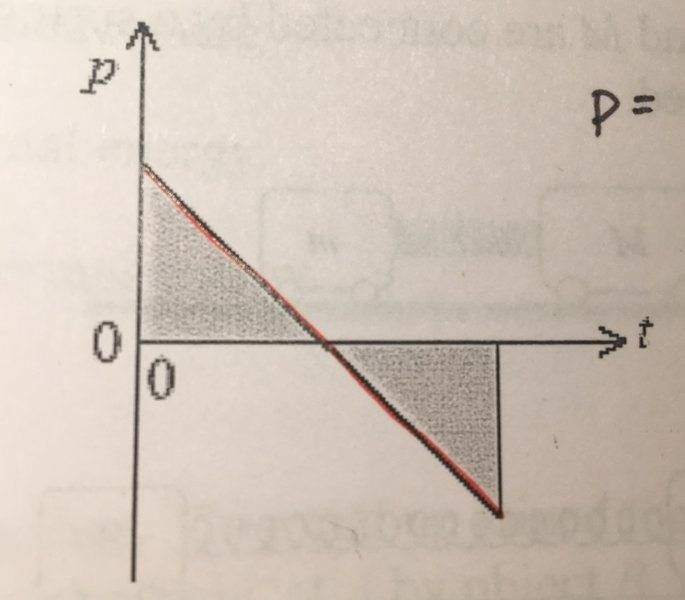# Ball-Wall collision problem

• PhysicsIsKillingMe

#### PhysicsIsKillingMe

Problem goes: A rubber ball, traveling in a horizontal direction, strikes a vertical wall. It rebounds at right angles to the wall. The graph below illustrates the variation of the ball’s momentum p with time t when the ball is in contact with the wall.Which of the following statements is true?

A) The shaded area is equal to the force exerted by the wall on the ball.
B) The shaded area is equal to the force exerted by the ball on the wall.
C) The gradient is equal to the force exerted by the wall on the ball.
D) The gradient is equal to the force exerted by the ball on the wall.

The right answer is C. I understand how the gradient in any momentum vs time graph is the force, but I don’t understand why it’s by the wall on the ball instead of the ball on the wall.

#### Attachments

•